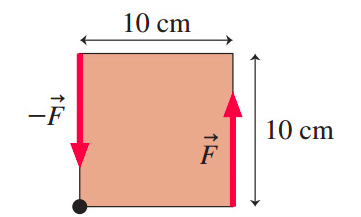# Problem: In the figure, what magnitude force provides 2.0 N•m net torque about the axle? Express your answer with the appropriate units.

###### FREE Expert Solution

Torque:

$\overline{){\mathbit{\tau }}{\mathbf{=}}{\mathbit{r}}{\mathbit{F}}}$

79% (224 ratings)###### Problem Details

In the figure, what magnitude force provides 2.0 N•m net torque about the axle? Express your answer with the appropriate units.